PSABPP3X - Student Facing Task---Acc7.5 Lesson 19 Fitting a Line to Data (8.SP.A.1, 8.SP.A.2)
Part A)

Here is a scatter plot that shows weights and fuel efficiencies of 20 different types of cars.

If a car weighs 1,750 kg, would you expect its fuel efficiency to be closer to 22 mpg or to 28 mpg?Select one:
Part B)

Part A)

Here is a table that shows weights and prices of 20 different diamonds.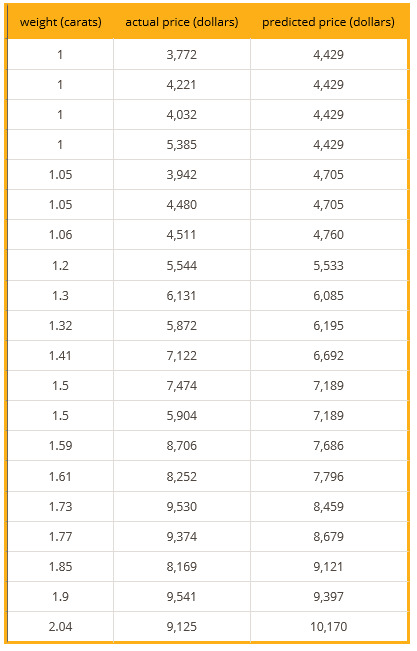The scatter plot shows the prices and weights of the 20 diamonds together with the graph of y=5,520x-1,091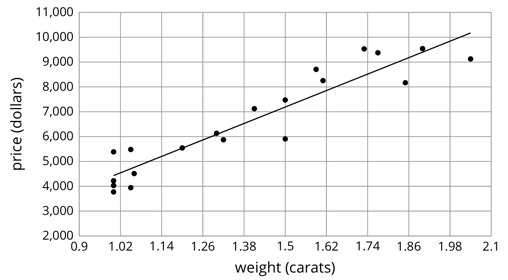The function described by the equation y=5,520x-1,091 is a model of the relationship between a diamond’s weight and its price.

This model predicts the price of a diamond from its weight. These predicted prices are shown in the third column of the table.

Two diamonds that both weigh 1.5 carats have different prices. What are their prices? How can you see this in the table? How can you see this in the graph?

Part B)

The model predicts that when the weight is 1.5 carats, the price will be \$7,189. How can you see this in the graph? How can you see this using the equation?

Part C)

One of the diamonds weighs 1.9 carats. What does the model predict for its price?

Type your answer below as a number (example: 5, 3.1, 4 1/2, or 3/2):
Part D)

How does that compare to the actual price?

Part E)

Find a diamond for which the model makes a very good prediction of the actual price. How can you see this in the table? In the graph?

Part F)

Find a diamond for which the model’s prediction is not very close to the actual price. How can you see this in the table? In the graph?

Part A)

Here is a scatter plot that shows lengths and widths of 20 different left feet.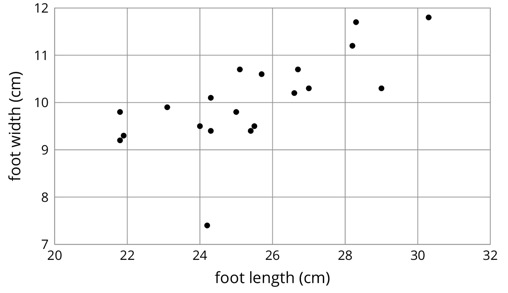Estimate the widths of the longest foot and the shortest foot.

Width of longest foot:

Part B)

Width of shortest foot:

Part C)

Estimate the lengths of the widest foot and the narrowest foot.

Length of widest foot:

Part D)

Length of narrowest foot:

Part E)

Here is the same scatter plot together with the graph of a model for the relationship between foot length and width.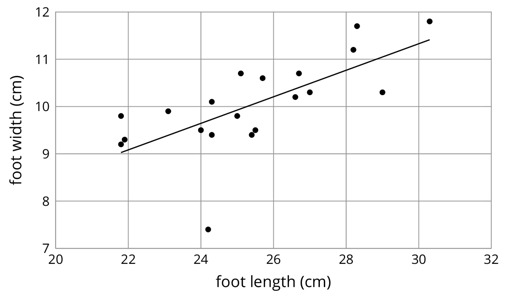Circle the data point that seems weird when compared to the model.

Draw your graph on paper, take a picture, and upload it using the upload icon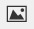If you do not have the ability to upload an image of your work, type "Graph is on paper."